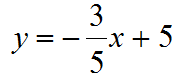7 Questions | Total Attempts: 474SettingsFind the gradient of a line from equations in different forms. Where gradient is not an integer, write it as a proper or improper fraction, ie 0. 5 = 1/2 or 3. 5 = 7/2.

• 1.
If the equation above is graphed, what will the gradient be?
• 2.
If the equation above is graphed, what will the gradient be?
• 3.
If the equation above is graphed, what will the gradient be?
• 4.
If the equation above is graphed, what will the gradient be?
• 5.
If the equation above is graphed, what will the gradient be?
• 6.
If the equation above is graphed, what will the gradient be?
• 7.
If the equation above is graphed, what will the gradient be?
• 8.
If the equation above is graphed, what will the gradient be?
• 9.
If the equation above is graphed, what will the gradient be?
• 10.
If the equation above is graphed, what will the gradient be?
• 11.
If the equation above is graphed, what will the gradient be?
• 12.
If the equation above is graphed, what will the gradient be?
• 13.
If the equation above is graphed, what will the gradient be?
• 14.
If the equation above is graphed, what will the gradient be?
• 15.
If the equation above is graphed, what will the gradient be?
• 16.
If the equation above is graphed, what will the gradient be?
• 17.
If the equation above is graphed, what will the gradient be?
• 18.
If the equation above is graphed, what will the gradient be?
• 19.
If the equation above is graphed, what will the gradient be?
• 20.
If the equation above is graphed, what will the gradient be?
• 21.
If the equation above is graphed, what will the gradient be?
• 22.
If the equation above is graphed, what will the gradient be?
• 23.
If the equation above is graphed, what will the gradient be?
• 24.
If the equation above is graphed, what will the gradient be?
• 25.
If the equation above is graphed, what will the gradient be?
Related TopicsBack to top